• 一、数据标准化(归一化)首先，数据标准化处理主要包括数据同趋化处理(中心化处理)和无量纲化处理。同趋化处理主要解决不同性质数据问题，对不同性质指标直接加总不能正确反映不同作用力的综合结果，须先考虑改变逆...


一、数据标准化(归一化)
首先，数据标准化处理主要包括数据同趋化处理(中心化处理)和无量纲化处理。同趋化处理主要解决不同性质数据问题，对不同性质指标直接加总不能正确反映不同作用力的综合结果，须先考虑改变逆指标数据性质，使所有指标对测评方案的作用力同趋化，再加总才能得出正确结果。无量纲化处理主要为了消除不同指标量纲的影响，解决数据的可比性，防止原始特征中量纲差异影响距离运算(比如欧氏距离的运算)。它是缩放单个样本以具有单位范数的过程，这与标准化有着明显的不同。简单来说，标准化是针对特征矩阵的列数据进行无量纲化处理，而归一化是针对数据集的行记录进行处理，使得一行样本所有的特征数据具有统一的标准，是一种单位化的过程。即标准化会改变数据的分布情况，归一化不会，标准化的主要作用是提高迭代速度，降低不同维度之间影响权重不一致的问题。
数据标准化(归一化)的方法有很多种，常用的有"最小-最大标准化"、"Z-score标准化"和"按小数定标标准化"等等。经过标准化处理后，原始数据均转换为无量纲化指标测评值，即各指标值都处于同一个数量级别上，可以进行综合测评分析。
这里介绍常用的三种常见的转换方法：
1. 极值法(区间缩放法)
线性比例变换法：正向指标：y = (x)/(max)，即新数据=(原数据)/(最大值)。负向指标：y = (min)/(x)，即新数据=(最小值)/(原数据)。
极差变换法：正向指标：y = (x- min)/(max - min)，即新数据=(原数据-最小值)/(最大值-最小值)。负向指标：y = (max - x)/(max - min)，即新数据=(最大值-原数据)/(最大值-最小值)。
使用这种方法的目的包括：
(1)0-1标准化，又称最大值-最小值标准化，核心要义是将原始指标缩放到0~1之间的区间内，但不改变原始数据的分布(2)对于方差非常小的属性可以增强其稳定性；(3)维持稀疏矩阵中为0的条目。
2. 比重法
L2正则化：y = x/sqrt(Σx^2)，即新数据=(原数据)/sqrt(平方和)，被称为L2正则转换。
正则化则是通过范数规则来约束特征属性，通过正则化我们可以降低数据训练处来的模 型的过拟合可能，和机器学习中所讲述的L1、L2正则的效果一样。在进行正则化 操作的过程中，不会改变数据的分布情况，但是会改变数据特征之间的相关特性。
3. 标准化Z-score：y = (x - mean)/σ，基于原始数据的均值(mean)和标准(standard deviation)进行数据的标准化，经过处理的数据符合标准正态分布，即均值为0，标准差为1。
4. 二值化
对于定量数据(特征取值连续)，根据给定的阈值将其进行转换，如果大于阈值赋值为1，否则赋值为0；对于定性数据(特征取值离散，也有可能是文本数据)，根据给定规则将其进行转换，符合规则的赋值为1，否则赋值为0。
二、特征转换
特征转换主要指将原始数据中的字段数据进行转换操作，从而得到适合进行算法 模型构建的输入数据(数值型数据)，在这个过程中主要包括但不限于以下几种数据的处理：文本数据转换为数值型数据、缺省值填充、定性特征属性哑编码、定量特征属性二值化、特征标准化与归一化。
1.文本特征属性转换：机器学习的模型算法均要求输入的数据必须是数值型的，所以对于文本类型的特征属性，需要进行文本数据转换，也就是需要将文本数据转换为数值型数据。常用方式如下：词袋法(BOW/TF)、TF-IDF(Term frequency-inverse document frequency)、HashTF、Word2Vec(主要用于单词的相似性考量)。
2.缺省值填充：缺省值是数据中最常见的一个问题，处理缺省值有很多方式，主要包括以下四个 步骤进行缺省值处理：确定缺省值范围->去除不需要的字段->填充缺省值内容->重新获取数据。
3.哑编码(OneHotEncoder)：也称哑变量处理，对于定性的数据(也就是分类的数据)，可以采用N位的状态寄存器来对N个状态进行编码，每个状态都有一个独立的寄存器位，并且在任意状态下只有一位有效；是一种常用的将特征数字化的方式。比如有一个特征属性:['male','female']，那么male使用向量[1,0]表示，female使用[0,1]表。
公众号推荐：数据思践
数据思践公众号记录和分享数据人思考和践行的内容与故事。《数据科学与人工智能》公众号推荐朋友们学习和使用Python语言，需要加入Python语言群的，请扫码加我个人微信，备注【姓名-Python群】，我诚邀你入群，大家学习和分享。关于Python语言，有任何问题或者想法，请留言或者加群讨论。


展开全文• 数据分析-数据平滑处理 数据平滑处理 在做数据分析时, 由于数据的噪声太多, 需要对数据进行数据平滑处理. 通常包含有降噪/拟合等操作. 降噪的功能在于去除额外的影响因素. 拟合的目的在于数学模型化,可以通过更多的...
数据分析-数据平滑处理
数据平滑处理
在做数据分析时, 由于数据的噪声太多, 需要对数据进行数据平滑处理. 通常包含有降噪/拟合等操作. 降噪的功能在于去除额外的影响因素. 拟合的目的在于数学模型化,可以通过更多的数学方法识别曲线特征.
降噪手段: 卷积运算
# 通常卷积核的选取API
con_core = np.hanning(8)
con_core /= con_core.sum()
# 卷积API
np.convolve(samples, con_core, 'valid')

数学模型化: 多项式拟合
# 通过原函数曲线(x,y)/最高次数 得到 拟合多项式系数
P = np.polyfit(x, y, n)
# 通过多项式系数与x的值,求对应的多项式函数值.
y = np.polyval(P, x)
# 通过原函数求导函数
Q = np.polyder(P)
# 求多项式的根
X = np.roots(Q)
# 求两个多项式函数的差函数. 可以通过该方法求取两个多项式
# 函数的交点
Q = np.polysub(P1, P2)

符号数组
sign函数可以把样本数组变成对应的符号数组, 所有正数变为1, 负数变为-1, 0还是0.
ary = np.sign(源数组)

绘制 净额成交量(OBV)
若相比上一天的收盘价上涨,则为正成交量; 若比上一天的收盘价下跌,则为负成交量.
成交量可以反映市场对某支股票的人气, 成交量是一支股票上涨的能量. 一般情况下股票上涨往往需要较大的成交量,而下跌时则不然.
案例: 绘制OBV柱状图
import numpy as np
import matplotlib.pyplot as mp
import datetime as dt
import matplotlib.dates as md

def dmy2ymd(dmy):
dmy = str(dmy, encoding='utf-8')
date = dt.datetime.strptime(
dmy, '%d-%m-%Y').date()
s = date.strftime("%Y-%m-%d")
return s

# 加载文件
'../data/bhp.csv', delimiter=',',
usecols=(1, 6, 7), unpack=True,
dtype='M8[D], f8, f8',
converters={1: dmy2ymd})

# 获取相比上一天股价是否上涨
diff_closing_prices = np.diff(closing_prices)
# 获取相对应的符号数组
sign_closing_prices = np.sign(diff_closing_prices)
# 绘制每天的成交量
obvs = volumes[1:] * sign_closing_prices

# 绘制净额成交量柱状图
mp.figure('OBV', facecolor='lightgray')
mp.title('OBV', fontsize=18)
mp.xlabel('Dates', fontsize=14)
mp.ylabel('Volumes', fontsize=14)
# 整理x轴刻度定位器
ax = mp.gca()
ax.xaxis.set_major_locator(
md.WeekdayLocator(byweekday=md.MO))
ax.xaxis.set_major_formatter(
md.DateFormatter('%d %b %Y'))
ax.xaxis.set_minor_locator(md.DayLocator())

mp.tick_params(labelsize=10)
mp.grid(linestyle=':', axis='y')
dates = dates[1:].astype(md.datetime.datetime)

mp.bar(dates, obvs, 1.0, color='dodgerblue',
edgecolor='white', label='OBV')

mp.gcf().autofmt_xdate()
mp.legend()
mp.show()

数组预处理函数
# array 源数组
# 条件序列:  [a<60, a==60, a>60]
# 取值序列:  [-1,   0,     1   ]
np.piecewise(array, 条件序列, 取值序列)

测试:
a = np.array([23, 94, 65, 23, 84, 56, 23])
d = np.piecewise(
a,
[(20 < a) & (a < 60), a == 60, a > 60],
[-1, 0, 1])
print(d)

矢量化
矢量化指的是用数组代理标量来操作数组里的每个元素.
numpy提供了vectorize函数, 可以把处理标量的函数矢量化. 经过vectorize函数矢量化处理过后将会返回一个矢量函数, 该函数可以直接处理ndarray数组.
案例:
import numpy as np
import math as m

def foo(x, y):
return m.sqrt(x**2 + y**2)

x, y = 3, 4
print(foo(x, y))
x = np.array([3, 4, 5, 6])
y = np.array([4, 5, 6, 7])
# z = foo(x, y)  错误
# 把foo函数矢量化处理
foo_v = np.vectorize(foo)
print(foo_v(x, y))

numpy提供了frompyfunc函数, 也可以完成vectorize相同的功能
# 使用frompyfunc方法矢量化函数
# foo需要2个参数, 最终将会有1个返回值
foo_f = np.frompyfunc(foo, 2, 1)
print(foo_f(x, y))

矩阵
numpy.matrix类型用来描述矩阵. 该类继承自numpy.ndarray. 任何多维数组的操作,对矩阵同样有效. 但是作为子类矩阵又结合了自身的特点,做了必要的扩充,比如乘法计算/矩阵求逆等等.
矩阵的创建
# ary: 任何可以被解释为矩阵的二维容器
# copy: 如果copy的值为True(缺省), 所得到的矩阵对象与参
# 数中源容器各自拥有独立的数据拷贝.否则,共享同一份数据
numpy.matrix(ary, copy=True)

# ary: 任何可以被解释为矩阵的二维容器
# 默认copy=False
numpy.mat(ary)

# str: 字符串矩阵拼块规则
#      '1 2 3;4 5 6;7 8 9'
np.mat(str)

矩阵的乘法运算
'''
a * b
矩阵的乘法:a矩阵的每一行分别乘以b矩阵的每一列
结果矩阵的行数与a的行数一致, 结果矩阵的列数与b的列数一致
'''
e = np.mat('1 2 6; 3 5 7; 4 8 9')
print(e * e)

矩阵的逆矩阵
若两个矩阵A/B满足: AB = BA = E (单位矩阵),则 A/B互为逆矩阵.
单位矩阵: 矩阵的主对角线值为1, 其他位置值为0的矩阵.
e = np.mat(..)
print(e.I)  #逆矩阵

案例 : 春游, 去程做大巴, 小孩票价3元, 家长票价3.2元, 一共花了118.4; 回程做火车, 小孩3.5, 家长3.6, 花了135.2; 求小孩与家长的人数.
\left[ \begin{array}{ccc} x & y \end{array} \right] \times \left[ \begin{array}{ccc} 3 & 3.5 \ 3.2 & 3.6 \ \end{array} \right]
\left[ \begin{array}{ccc} 118.4 & 135.2 \end{array} \right]
斐波那契数列
1 1 2 3 5 8 13 ...

1 1  1 1  1 1  1 1
X  1 0  1 0  1 0  1 0
---------------------------------------------
1 1  2 1  3 2  5 3
1 0  1 1  2 1  3 2  ...

numpy通用函数
加法通用函数
np.add(a, b)			# 两数组相加

案例:
a = np.arange(1, 7)
print(a)

除法通用函数
# 真除
a / b
np.true_divide(a, b)
np.divide(a, b)
# 地板除
np.floor_divide(a, b)
np.ceil(a / b)	# 天花板除
np.trunc(a / b) # 截断除

import numpy as np

a = np.array([20, 20, -20, -20])
b = np.array([3, -3, 6, -6])
print(a)
print(b)
# 开始测试
print(np.true_divide(a, b))
print(np.divide(a, b))

print(np.floor_divide(a, b))
print(np.ceil(a / b))
print(np.trunc(a / b))

三角函数通用函数
np.sin()  np.cos()

傅里叶定理
傅里叶说过, 任何周期函数都可以由多个不同振幅/频率/相位的正弦函数叠加而成.
合成方波
一个方波由如下参数的正弦波叠加而成:
y = 4\pi \times sin(x) \ y = \frac{4}{3}\pi \times sin(3x) \ y = \frac{4}{5}\pi \times sin(5x) \ y = \frac{4}{7}\pi \times sin(7x) \ … \ y = \frac{4}{2n-1}\pi \times sin((2n-1)x) \
案例:
import numpy as np
import matplotlib.pyplot as mp

x = np.linspace(-2 * np.pi, 2 * np.pi, 1000)
# 根据公式搞出来3条曲线
y1 = 4 * np.pi * np.sin(x)
y2 = 4 / 3 * np.pi * np.sin(3 * x)
y3 = 4 / 5 * np.pi * np.sin(5 * x)

# 使用循环控制叠加波的数量
n = 1000
y = np.zeros(1000)
for i in range(1, n + 1):
y += 4 / (2 * i - 1) * np.pi * \
np.sin((2 * i - 1) * x)

mp.figure('SIN', facecolor='lightgray')
mp.title('SIN', fontsize=18)
mp.xlabel('X', fontsize=14)
mp.ylabel('Y', fontsize=14)
mp.tick_params(labelsize=10)

mp.plot(x, y1, label='y1')
mp.plot(x, y2, label='y2')
mp.plot(x, y3, label='y3')
mp.plot(x, y, label='y')

mp.legend()
mp.show()

位运算通用函数
位异或
c = a ^ b
c = np.bitwise_xor(a, b)

按位异或可以很方便的判断两个数据是否同号
a = np.array([4, -6, 7, -3, -4, 2])
b = np.array([-2, -8, 2, -5, 3, -4])

print(a)
print(b)
print(a ^ b)
print(np.bitwise_xor(a, b))
# where找到符合条件的元素下标 (异号)
print(np.where((a ^ b) < 0))

位与
e = a & b
e = np.bitwise_and(a, b)

利用位与运算计算某个数字是否是2的幂
'''
1  2^0  00001    0  00000
2  2^1  00010    1  00001
4  2^2  00100    3  00011
8  2^3  01000    7  00111
'''
print('-' * 40)
d = np.arange(1, 20)
print(d)
e = np.bitwise_and(d, d - 1)
print(e)

位或 / 位反 / 移位
# 位或操作
np.bitwise_or(a, b)
# 位反操作 (1变0, 0变1)
np.bitwise_not(a)
# 移位操作
np.left_shift(array, 1)		#每个元素左移1位 (乘2)
np.right_shift(array, 1)	#每个元素右移1位 (除2)

numpy提供的线性代数模块(linalg)
逆矩阵和广义逆矩阵
如果一个方阵A与方阵B的乘积是单位矩阵,则AB互为逆矩阵.
np.linalg.inv(A)  # 返回A方阵的逆矩阵

如果A不是方阵, A的逆矩阵则称为广义逆矩阵.
np.linalg.pinv(A) # 返回矩阵A的广义逆矩阵

d = A.I # 既可以返回逆矩阵也可以返回广义逆矩阵

线性方程求解与线性拟合
# 求解线性方程组
c = np.linalg.solve(a, b)
# 线性拟合(求出误差最小的结果矩阵)
c = np.linalg.lstsq(a, b)

\begin{cases} x -2y+z=0\ 2y-8z=8\ -4x+5y+9z=-9 \end{cases}
展开全文• ## python数据过滤 平滑

千次阅读 2019-11-22 12:57:42
https://www.cnblogs.com/0820LL/p/10363635.html 这篇文章写的挺好，个人用可以
https://www.cnblogs.com/0820LL/p/10363635.html
这篇文章写的挺好，个人用可以
展开全文• python 数据、曲线平滑处理——方法总结Savitzky-Golay 滤波器实现曲线平滑插值法对折线进行平滑曲线处理基于Numpy.convolve实现滑动平均滤波数据平滑处理——log()和exp()函数 问题描述： 在寻找曲线的波峰、波谷时...


python 数据、曲线平滑处理——方法总结
Savitzky-Golay 滤波器实现曲线平滑插值法对折线进行平滑曲线处理基于Numpy.convolve实现滑动平均滤波数据平滑处理——log()和exp()函数

问题描述：

在寻找曲线的波峰、波谷时，由于数据帧数多的原因，导致生成的曲线图噪声很大，不易寻找规律。如下图：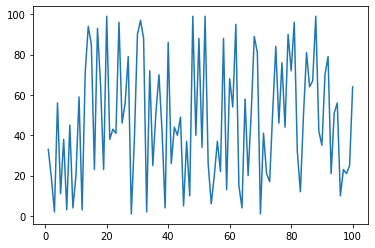由于高频某些点的波动导致高频曲线非常难看，为了降低噪声干扰，需要对曲线做平滑处理，让曲线过渡更平滑。常见的对曲线进行平滑处理的方法包括： Savitzky-Golay 滤波器、插值法等。
Savitzky-Golay 滤波器实现曲线平滑
对曲线进行平滑处理，通过Savitzky-Golay 滤波器，可以在scipy库里直接调用，不需要再定义函数。
代码语法：
python中Savitzky-Golay滤波器调用如下：
y_smooth = scipy.signal.savgol_filter(y,53,3)
# 亦或
y_smooth2 = savgol_filter(y, 99, 1, mode= 'nearest')

# 备注：
y：代表曲线点坐标（x,y）中的y值数组
window_length：窗口长度，该值需为正奇整数。例如：此处取值53
k值：polyorder为对窗口内的数据点进行k阶多项式拟合，k的值需要小于window_length。例如：此处取值3
mode：确定了要应用滤波器的填充信号的扩展类型。（This determines the type of extension to use for the padded signal to which the filter is applied. ）

调参规律：
现在看一下window_length和k这两个值对曲线的影响。
（1）window_length对曲线的平滑作用： window_length的值越小，曲线越贴近真实曲线；window_length值越大，平滑效果越厉害（备注：该值必须为正奇整数）。
（2）k值对曲线的平滑作用： k值越大，曲线越贴近真实曲线；k值越小，曲线平滑越厉害。另外，当k值较大时，受窗口长度限制，拟合会出现问题，高频曲线会变成直线。
典型范例：
# 用于生成问题描述中示例曲线的代码如下：
import numpy as np

Size = 100
x = np.linspace(1, Size,Size)

data = np.random.randint(1, Size, Size)
print(data)
>>>
array([33, 19,  2, 56, 11, 38,  3, 45,  4, 20, 59,  3, 71, 94, 85, 23, 93,
65, 23, 99, 38, 43, 41, 96, 46, 56, 79,  1, 38, 90, 97, 88,  2, 72,
25, 51, 70, 42,  4, 86, 26, 44, 40, 49,  5, 37, 10, 99, 40, 88, 34,
99, 26,  6, 20, 37, 22, 88, 13, 68, 54, 95, 15,  4, 58, 20, 51, 89,
81,  1, 41, 21, 17, 52, 84, 46, 76, 44, 90, 72, 96, 32, 12, 50, 81,
64, 67, 99, 42, 35, 70, 79, 21, 51, 56, 10, 23, 21, 25, 64])

# 可视化图线
plt.plot(x, data)

# 使用Savitzky-Golay 滤波器后得到平滑图线
from scipy.signal import savgol_filter

y = savgol_filter(data, 5, 3, mode= 'nearest')
# 可视化图线
plt.plot(x, y, 'b', label = 'savgol')

下图所示为经过Savitzky-Golay 滤波器处理的示例曲线效果图：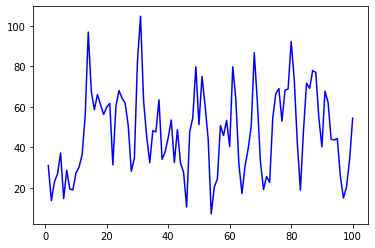原理剖析：
在scipy官方帮助文档里可以看到关于Savitzky-Golay 滤波器中关于savgol_filter()函数的详细说明。
以下是关于Savitzky-Golay平滑滤波的简单介绍（引至博客：Python 生成曲线进行快速平滑处理）：
Savitzky-Golay平滑滤波是光谱预处理中的常用滤波方法，其 核心思想：是对一定长度窗口内的数据点进行k阶多项式拟合，从而得到拟合后的结果。 对它进行离散化处理后，Ｓ-Ｇ 滤波其实是一种移动窗口的加权平均算法，但是其加权系数不是简单的常数窗口，而是通过在滑动窗口内对给定高阶多项式的最小二乘拟合得出。
Savitzky-Golay平滑滤波被广泛地运用于数据流平滑除噪，是一种在时域内基于局域多项式最小二乘法拟合的滤波方法。这种滤波器的 最大特点：在滤除噪声的同时可以确保信号的形状、宽度不变。
使用平滑滤波器对信号滤波时，实际上是拟合了信号中的低频成分，而将高频成分平滑出去了。 如果噪声在高频端，那么滤波的结果就是去除了噪声，反之，若噪声在低频段，那么滤波的结果就是留下了噪声。
总之，平滑滤波是光谱分析中常用的预处理方法之一。用Savitzky-Golay方法进行平滑滤波，可以提高光谱的平滑性，并降低噪音的干扰。S-G平滑滤波的效果，随着选取窗宽不同而不同，可以满足多种不同场合的需求。
参考链接：Savitzky-Golay平滑滤波的python实现
插值法对折线进行平滑曲线处理
实现所需的库： numpy、scipy、matplotlib
插值法的常见实现方法：
nearest：最邻近插值法zero：阶梯插值slinear：线性插值quadratic、cubic：2、3阶B样条曲线插值
拟合和插值的区别：
1、插值：简单来说，插值就是根据原有数据进行填充，最后生成的曲线一定过原有点。
2拟合：拟合是通过原有数据，调整曲线系数，使得曲线与已知点集的差别（最小二乘）最小，最后生成的曲线不一定经过原有点。
代码语法：
通过执行from scipy.interpolate import make_interp_spline，导入make_interp_spline模块，之后调用make_interp_spline(x, y)(x_smooth)函数实现。
官方帮助文档：scipy.interpolate.make_interp_spline
典型范例：
import numpy as np
from matplotlib import pyplot as plt
from scipy.interpolate import make_interp_spline

x = np.array([6, 7, 8, 9, 10, 11, 12])
y = np.array([1.53E+03, 5.92E+02, 2.04E+02, 7.24E+01, 2.72E+01, 1.10E+01, 4.70E+00])
x_smooth = np.linspace(x.min(), x.max(), 300)  # np.linspace 等差数列,从x.min()到x.max()生成300个数，便于后续插值
y_smooth = make_interp_spline(x, y)(x_smooth)
plt.plot(x_smooth, y_smooth)
plt.show()

上述执行代码的效果如下：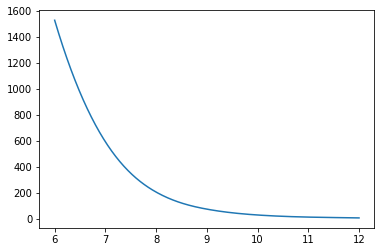参考链接：python利用插值法对折线进行平滑曲线处理
基于Numpy.convolve实现滑动平均滤波
滑动平均概念：
滑动平均滤波法 （又称：递推平均滤波法），它把连续取N个采样值看成一个队列 ，队列的长度固定为N ，每次采样到一个新数据放入队尾，并扔掉原来队首的一次数据(先进先出原则) 。把队列中的N个数据进行算术平均运算，就可获得新的滤波结果。
N值的选取：流量，N=12；压力：N=4；液面，N=4 ~ 12；温度，N=1~4
滑动平均的优缺点：
优点： 对周期性干扰有良好的抑制作用，平滑度高，适用于高频振荡的系统。
缺点： 灵敏度低，对偶然出现的脉冲性干扰的抑制作用较差，不易消除由于脉冲干扰所引起的采样值偏差，不适用于脉冲干扰比较严重的场合，比较浪费RAM 。
滑动平均的数学原理：
滑动平均滤波法计算类似一维卷积的工作原理，滑动平均的N就对应一维卷积核大小（长度）。 不过区别在于：
（1）步长会有些区别，滑动平均滤波法滑动步长为1，而一维卷积步长可以自定义； （2）一维卷积的核参数是需要更新迭代的，而滑动平均滤波法核参数都是1。
我们应该怎么利用这个相似性呢？其实也很简单，只需要把一维卷积核大小（长度）和N相等，步长设置为1，核参数都初始为1就可以了。由于一维卷积计算速度快，因此我们可以使用一维卷积来快速高效地实现这个功能。
代码语法：
通过Numpy库中的convolve()函数可以实现这些功能。
def np_move_avg(a,n,mode="same"):
return(np.convolve(a, np.ones((n,))/n, mode=mode))

Numpy.convolve函数：（numpy.convolve函数官方文档）
numpy.convolve(a, v, mode=‘full’)  # 返回a和v的离散线性卷积。

参数说明：
a:(N,)输入的第一个一维数组v:(M,)输入的第二个一维数组mode:{‘full’, ‘valid’, ‘same’}参数可选，该参数指定np.convolve函数如何处理边缘。

mode可能的三种取值情况： full’　默认值，返回每一个卷积值，长度是N+M-1,在卷积的边缘处，信号不重叠，存在边际效应。 ‘same’　返回的数组长度为max(M, N),边际效应依旧存在。 ‘valid’ 　返回的数组长度为max(M,N)-min(M,N)+1,此时返回的是完全重叠的点。边缘的点无效。

和一维卷积参数类似，a就是被卷积数据，v是卷积核大小。
原理剖析：
滑动平均值是卷积数学运算的一个例子。对于滑动平均值，沿着输入滑动窗口并计算窗口内容的平均值。对于离散的1D信号，卷积是相同的，除了代替计算任意线性组合的平均值，即将每个元素乘以相应的系数并将结果相加。那些系数，一个用于窗口中的每个位置，有时称为卷积核。现在，N值的算术平均值是

(

x

1

+

x

2

+

.

.

.

+

x

N

)

/

N

(x_1 + x_2 + ... + x_N) / N

，所以相应的内核是

(

1

/

N

,

1

/

N

,

.

.

.

,

1

/

N

)

(1/N, 1/N, ..., 1/N)

，这正是我们通过使用得到的np.ones((N,))/N。
np.convolve函数中通过mode参数指定如何处理边缘。下面是一个说明模式不同取值之间差异的图：
import numpy as np
import matplotlib.pyplot as plt

def np_move_avg(a,n,mode="same"):
return(np.convolve(a, np.ones((n,))/n, mode=mode))

modes = ['full', 'same', 'valid']

for m in modes:
plt.plot(np_move_avg(np.ones((200,)), 50, mode=m))

plt.axis([-10, 251, -.1, 1.1])

plt.legend(modes, loc='lower center')

plt.show()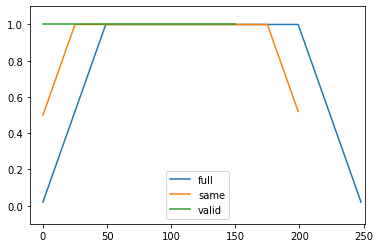参考链接： [开发技巧]·Python极简实现滑动平均滤波（基于Numpy.convolve） numpy中的convolve的理解
典型范例：
# 实现数据可视化中的数据平滑
import numpy as np
import matplotlib.pylab as plt

'''
其它的一些知识点：
raise：当程序发生错误，python将自动引发异常，也可以通过raise显示的引发异常
一旦执行了raise语句，raise语句后面的语句将不能执行
'''

def moving_average(interval, windowsize):
window = np.ones(int(windowsize)) / float(windowsize)
re = np.convolve(interval, window, 'same')
return re

def LabberRing():
t = np.linspace(-4, 4, 100)   # np.linspace 等差数列,从-4到4生成100个数
print('t=', t)
# np.random.randn 标准正态分布的随机数，np.random.rand 随机样本数值
y = np.sin(t) + np.random.randn(len(t)) * 0.1   # 标准正态分布中返回1个，或者多个样本值
print('y=', y)

plt.plot(t, y, 'k')     # plot(横坐标，纵坐标， 颜色)

y_av = moving_average(y, 10)
plt.plot(t, y_av, 'b')
plt.xlabel('Time')
plt.ylabel('Value')
# plt.grid()网格线设置
plt.grid(True)
plt.show()
return

LabberRing()  # 调用函数

黑色曲线为原始折线，蓝色曲线为通过np.convolve函数进行平滑处理后的曲线效果。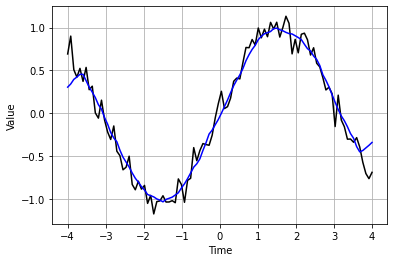参考链接：数据可视化中的数据平滑
数据平滑处理——log()和exp()函数
log()函数的使用就像是将一个数据压缩到了一个区间，与数据的标准化类似。
在数据预处理时，引入log()函数的优点：
1、在数据预处理时首先可以对偏度比较大的数据用log()函数进行转化，使其更加服从高斯分布，此步处理可能会使我们后续的分类结果得到一个更好的结果。
2、在数据、曲线平滑处理时，同样需要使用log()函数，从而避免复值的问题 ，否则，将导致模型的结果总是达不到一定的标准。（备注： 复值指一个自变量对应多个因变量）
下面再说说它的 逆运算 exp()函数。
由于前面使用过log()将数据进行了压缩，所以最后需要记得将预测出的平滑数据进行一个还原，而还原过程就是log()函数的逆运算exp()函数。
常用的数学表达式：

l

o

g

1

p

=

l

o

g

(

x

+

1

)

即

：

l

n

(

x

+

1

)

log1p = log(x+1)即：ln(x+1)

e

x

p

m

1

=

e

x

p

(

x

)

−

1

expm1 = exp(x)-1

log1p函数有它存在的意义，即保证了x数据的有效性，当x很小时（如 两个数值相减后得到

x

=

1

0

−

16

x = 10^{-16}

），由于太小超过数值有效性，用

l

o

g

(

x

+

1

)

log(x+1)

计算得到结果为0，换作log1p则计算得到一个很小却不为0的结果，这便是它的意义（好像是用泰勒公式来展开运算的，不确定）。 同样的道理对于expm1，当x特别小，就会急剧下降出现如上问题，甚至出现错误值。 在最开始看到这样的处理方式的时候，不是很理解包括为什么是逆运算（一下子没有想到），后来慢慢摸索就优点清晰了，比如为什么两这是逆运算（简单处理）：

l

o

g

x

logx

是e为底的对数，

e

x

e^x

是e为底的指数，根据对数的规则，再进行变换推导可以得到：

e

l

o

g

e

x

=

x

e^{log_e^x}=x

可以看到x经过对数的处理后，再经过指数处理再次得到x，这里对两者的逆运算做了简单的介绍。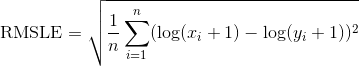另外RMSLE（均方根对数误差）会更多的惩罚欠拟合，所以在使用该误差定义时我们也可以用到上面的函数： np.loglp计算加一后的对数，其逆运算是np.expm1； 采用此误差函数时，可以先对原始数据做np.log1p，再使用RMSE。

参考链接：数据平滑处理——log1p()和exmp1()
展开全文• 主要为大家详细介绍了python利用插值法对折线进行平滑曲线处理，具有一定的参考价值，感兴趣的小伙伴们可以参考一下
• 效果展示 代码展示 import pandas as pd import matplotlib.pyplot as plt plt.subplot(2,2,2) pd.Series(accs_train, index=iters).ewm(span=10).mean().plot(label='EMA_train_accs') pd.Series(accs_train, ...机器学习 深度学习 人工智能 plt
• 平滑数据噪声的一个简单朴素的做法是，对窗口（样本）求平均，然后仅仅绘制出给定窗口的平均值，而不是所有的数据点。 import matplotlib.pyplot as plt import numpy as np def moving_average(interval, ...
• 我们对数据应用一些运算符，改变源点，以消除高频波动。 操作符的例子：scipy.signal.convolve、scipy.signal.medfilt、scipy.signal.savgol_filter等。 平滑算子是分布理论中具有特殊性质的平稳函数，用来建立一...
• Python数据处理】批量导出深度学习网络训练生成的event格式文件中的数据到同一excel表的不同sheet 上一篇blog已经把训练生成的event数据导入到excel中去，（笔者生成的event格式文件是训练深度学习网络生成的文件...
• GDAL是空间数据处理的开源包，支持多种数据格式的读写。遥感图像是一种带大地坐标的栅格数据，遥感图像的栅格模型包含以下两部分的内容： 栅格矩阵：由正方形或者矩形栅格点组成，每个栅格点所对应的数值为该点的像...
• python 数据、曲线平滑处理——方法总结 Savitzky-Golay 滤波器、make_interp_spline插值法和convolve滑动平均滤波
• ## Python 生成曲线进行快速平滑处理

万次阅读 多人点赞 2019-09-03 23:20:46
python函数： ... Savitzky-Golay 滤波器 ...Savitzky-Golay平滑滤波是光谱预处理中常用滤波方法，它的核心思想是对一定长度窗口内的数据点进行k阶多项式拟合，从而得到拟合后的结果。对它进行离散化处理后后，Ｓ-...
• 严重影响到数据挖掘建模的执行效率，甚至可能导致挖掘结果的偏差，所以进行数据清洗就显得尤为重要，数据清洗完成后接着进行或者同时进行数据集成、转换、规约等一系列的处理，该过程就是数据预处理。数据预处理一...
• 数据文件已经项目目录下，直接在IDE导入项目运行即可。（注：该项目是在jdk1.8环境下编译） 数据文件已经项目目录下，直接在IDE导入项目运行即可。（注：该项目是在jdk1.8环境下编译）
• 数据平滑处理 – log1p( ) 和 exmp1( ) 在数据预处理时首先可以对偏度比较大的数据用log1p函数进行转化，使其更加服从高斯分布，此步处理可能会使我们后续的分类结果得到一个更好的结果； 平滑处理很容易被忽略掉，...机器学习
• 平滑滤波是光谱分析中常用的预处理方法之一，处理的方法有很多，这里我们使用make_interp_spline插值法对折线图进行平滑处理。 官方帮助文档：scipy.interpolate.make_interp_spline 处理前的图 处理后的图 源码...matlab 插值法
• 几种常见的平滑算法 在计算语言模型的过程中，对于句子中的每一个字符或者词都需要一个非零的概率值，因为一旦存在一个概率为 0的结果，那么整个计算公式的结果都为 0，这种问题我们叫做数据匮乏（稀疏），所以...
• 线性平滑滤波，适用于消除高斯噪声，广泛应用于图像处理的减噪过程，对整幅图像进行加权平均，每一个像素点的值，都由其本身和邻域内的其他像素值经过加权平均后得到 def imgGaussianFilter(img,kernel): return ...高斯滤波 均值滤波 中值滤波
• 文章目录一、概述二、算法原理三、python代码实现3.1 构造模拟信号3.2 编写平滑处理代码3.3 展示算法效果 一、概述   通过采集仪和传感器采样得到的振动信号数据往往叠加有噪声信号。噪声信号除了有50Hz的工频干扰...算法
• python 平滑时间序列In time series analysis, the presence of dirty and messy data can alter our reasonings and conclusions. This is true, especially in this domain, because the temporal dependency ...java 人工智能 机器学习 leetcode
• 数据平滑：通常包含降噪、拟合等操作。降噪的功能在于去除额外的影响因素，拟合的目的在于数学模型化，可以通过更多的数学方法识别曲线的特征。 2.绘制两支股票的收益率 ---收益率 =（后一天的收盘价 - 前一天的...
• 装载自：... import numpy as np import pandas as pd def detect_outliers(data, threshold=3): """离群点检测""" mean_d = np.mean(data) std_d = np.std(data) outliers = [] ... for y in data:
• ## 数据预处理之python实现噪声处理

千次阅读 多人点赞 2020-03-18 10:35:18
数据集中的干扰数据（对场景描述不准确的数据） 2.噪声怎么产生的？举个例子：手机信号来自于基站发射的电磁波，有的地方比较强，有的地方比较弱。运营商的工程师会负责统计不同区域信号强弱来进行网络规划，工程师...
• 由于高频某些点的波动导致高频曲线非常难看，为了降低噪声干扰，需要对曲线做平滑处理，让曲线过渡更平滑，可以看出经过平滑处理后更明显去除噪声且更加美观。 1.滑动平均滤波 滑动平均滤波法（又称：递推平均滤波...人工智能 强化学习
• python+opencv图像处理之六：图像平滑处理 目录python+opencv图像处理之六：图像平滑处理一、图像平滑二、均值滤波三、中值滤波四、方框滤波五、高斯滤波 一、图像平滑 图像平滑是指受传感器和外界因素的影响，图像...计算机视觉 opencv
• python使用matplotlib.pyplot 画曲线，并平滑处理 import matplotlib.pyplot as plt list0 = ['数据'] plt.plot(list0) plt.show() 得到： 接下来平滑处理： 先导入： import scipy.signal 使用Savitzky-Golay...scipy
• 在用python绘图的时候，经常由于数据的原因导致画出来的图折线分界过于明显，因此需要对原数据绘制的折线进行平滑处理，本文介绍利用插值法进行平滑曲线处理： 实现所需的库 numpy、scipy、matplotlib 插值法...
• 1.高斯平滑 （1）高斯卷积核的构建 假设构造宽（列数）为、高（行数）为的高斯卷积算子，其中和均为奇数，锚点的位置在，步骤如下： 第一步：计算高斯矩阵。 其中 r，c代表位置索引，其中，，且r，c均为...OpenCV
• 2.12 真实数据的噪声平滑处理 前言：来自各种真实世界传感器的数据通常是不平滑和不干净的，也包含了一些我们不想在显示在图表或者图形的噪声。 1、操作步骤 （1）基础算法是基于滚动窗口模式（rolling window） （2...scipy numpy
• ## Python曲线平滑

千次阅读 2017-08-24 16:37:10
使用scipy.signal.medfilt函数可以对曲线进行平滑处理，第一个参数是要处理的数据，第二个参数一般是奇数，中间是数据本身，剩余为该点前后数据点，比如下面的3，代表取点本身以及前后各一个点的数据取中位数。...plot...

# python数据平滑处理python 订阅